# Social Services Spending and Health Outcomes¶

I recently read an interesting paper that looked at the relationship between social services spending and health outcomes across the OECD countries . The authors of this paper ran a few regressions using healthcare and social services spending as a percentage of GDP as the predictors of population health outcomes. Here's a summary of what they found:

In model 1, we found that health-service expenditures as a percentage of GDP were significantly associated with better health outcomes in only two of the five indicators (life expectancy and maternal mortality). In model 2, social expenditures as a percentage of GDP were significantly associated with better health outcomes in three of the five indicators (life expectancy, infant mortality and potential years of life lost) and with worse health outcomes in one of the indicators (low birth weight).

These findings fit with other research on the social determinants of health that suggests access to the healthcare system might only account for a small portion of overall health -- other things like income, education levels, and quality of public institutions might make larger contributions (although this is controversial) [2,3]. So if we can target some of those determinants through social services spending, it has the potential to be a very cost effective approach to improving health.

The authors in the above study used Potential Years of Life Lost (PYLL) as one of the main measures of population health. This metric doesn't include years lived with a disability (YLD), so I was interested in seeing how a more comprehensive measure of population health like the Disability Adjusted Life Year (YLLs + YLDs) affects this analysis. The Global Burden of Disease regularly conducts a study of global DALY burdens, so I dowloaded their 1990-2015 results. I also used per capita health and social services spending rather than spending as a percentage of GDP because I think the per capita figures are closer to what you want to measure.

The rest of this post looks at some of the relationships I found in the data. All the code is available in this IPython notebook, and the input data is available in a zipped file here.

# Getting the Data¶

I downloaded the 2015 Global Burden of Disease data from IHME's website here. The units are DALYs per 100,000 population, and it's age standardized across the countries. I also downloaded the OECD Social Services spending data from their website, with units of constant 2010 US dollars per capita, at purchasing power parity.

Below, I import the datasets and combine them into a single dataframe to use for the analysis.

In :
%matplotlib inline

import pandas as pd
import os
import glob
import matplotlib.pyplot as plt
import matplotlib
import numpy as np
#from scipy import stats

import statsmodels.formula.api as smf
import statsmodels.api as sm

matplotlib.style.use('ggplot')  #'ggplot' 'fivethirtyeight'  'seaborn-paper'
matplotlib.rcParams['figure.figsize'] = (11.0, 9.0)

pd.set_option('max_colwidth', 400)

In :
# 'Latvia', leave out because joined in July 2016
oecd_countries = ['Slovenia', 'France', 'Estonia', 'Switzerland', 'United States', 'Spain',
'Luxembourg', 'Israel', 'Sweden', 'Germany', 'Denmark', 'Australia',
'United Kingdom', 'Greece', 'Iceland', 'Chile', 'South Korea', 'Finland', 'Slovakia',
'Belgium', 'Japan', 'Mexico', 'Hungary', 'Austria', 'Italy', 'Portugal', 'Poland',
'Netherlands', 'Czech Republic', 'Ireland', 'New Zealand', 'Turkey', 'Norway', 'Canada']

#Contains all data 1990-2015

gbd_df = gbd_df[(gbd_df['cause'] == 'All causes') &
(gbd_df['location'].isin(oecd_countries)) &
(gbd_df['age'] == 'Age-standardized') &
(gbd_df['metric'] == "Rate") &
(gbd_df['measure'] == "DALYs (Disability-Adjusted Life Years)")]

gbd_df.rename(columns={'val': 'dalyrate'}, inplace=True)

gbd_df['yerr_upper'] = gbd_df['upper'] - gbd_df['dalyrate']
gbd_df['yerr_lower'] = gbd_df['dalyrate'] - gbd_df['lower']
gbd_df.reset_index(drop=True, inplace=True)

#Returns 34 countries
#gbd_df['year'].drop_duplicates()

Out:
measure location sex age cause metric year dalyrate upper lower yerr_upper yerr_lower
0 DALYs (Disability-Adjusted Life Years) Sweden Both Age-standardized All causes Rate 1990 22591.523826 25539.541245 20002.074540 2948.017418 2589.449286
1 DALYs (Disability-Adjusted Life Years) Czech Republic Both Age-standardized All causes Rate 1990 31761.321425 34892.755747 29069.197178 3131.434321 2692.124248
2 DALYs (Disability-Adjusted Life Years) Slovenia Both Age-standardized All causes Rate 1990 28762.376308 31857.034564 25995.537639 3094.658256 2766.838670
3 DALYs (Disability-Adjusted Life Years) Denmark Both Age-standardized All causes Rate 1990 26780.566573 29873.609543 24065.397680 3093.042970 2715.168893
4 DALYs (Disability-Adjusted Life Years) Canada Both Age-standardized All causes Rate 1990 23881.693184 26997.163892 21099.586785 3115.470707 2782.106400
In :
# Social expenditure data from 1990, 1995, 2000, 2005, and 2010.
# Nothing later, although there is through 2014 as a percent of GDP.
#https://stats.oecd.org/Index.aspx?DataSetCode=SOCX_AGG#

#Uncomment to view by Percentage of GDP:
#oecd_total_df = oecd_ss_df[(oecd_ss_df['UNIT'] == 'PCT_GDP') & (oecd_ss_df['Branch'] == 'Total')]
oecd_df = oecd_ss_df[(oecd_ss_df['UNIT'] == 'PPPVH') &
(oecd_ss_df['Branch'] == 'Total') &
(oecd_ss_df['Year'].isin([1990, 1995, 2000, 2005, 2010]))]
oecd_df.rename(columns={'Value': 'total_exp'}, inplace=True)

#Uncomment to view by Percentage of GDP:
#oecd_health_df = oecd_ss_df[(oecd_ss_df['UNIT'] == 'PCT_GDP') & (oecd_ss_df['Branch'] == 'Health')]
oecd_health_df = oecd_ss_df[(oecd_ss_df['UNIT'] == 'PPPVH') &
(oecd_ss_df['Branch'] == 'Health') &
(oecd_ss_df['Year'].isin([1990, 1995, 2000, 2005, 2010]))]

oecd_health_df.rename(columns={'Value': 'health_exp'}, inplace=True)

oecd_df = oecd_df.merge(oecd_health_df[['Country','Year', 'health_exp']], on=('Country', 'Year'))
#Remove health expenditure from total expenditure
oecd_df['social_exp'] = oecd_df['total_exp'] - oecd_df['health_exp']
#Add ratio of health to social
oecd_df['social_health_ratio'] = oecd_df['social_exp'] / oecd_df['health_exp']

oecd_df = oecd_df.loc[:, ['Country', 'Year','Source','Unit', 'Measure', 'total_exp', 'health_exp',
'social_exp','social_health_ratio']]

oecd_df['Country'] = oecd_df['Country'].replace(
['Slovak Republic', 'Korea' ], ['Slovakia', 'South Korea'])

oecd_df.columns = oecd_df.columns.str.lower()

#Drop nan rows:
oecd_df.dropna(axis='index', how='any', subset=['total_exp','social_exp','health_exp'], inplace=True)


Out:
country year source unit measure total_exp health_exp social_exp social_health_ratio
83 Luxembourg 2010 Public US Dollar Per head, at constant prices (2010) and constant PPPs (2010), in US dollars 19358.616 5127.791 14230.825 2.775235
82 Luxembourg 2005 Public US Dollar Per head, at constant prices (2010) and constant PPPs (2010), in US dollars 18251.951 4920.312 13331.639 2.709511
81 Luxembourg 2000 Public US Dollar Per head, at constant prices (2010) and constant PPPs (2010), in US dollars 13968.833 3613.952 10354.881 2.865251
103 Norway 2010 Public US Dollar Per head, at constant prices (2010) and constant PPPs (2010), in US dollars 12889.865 3289.478 9600.387 2.918514
29 Denmark 2010 Public US Dollar Per head, at constant prices (2010) and constant PPPs (2010), in US dollars 12109.237 2807.799 9301.438 3.312715
102 Norway 2005 Public US Dollar Per head, at constant prices (2010) and constant PPPs (2010), in US dollars 12325.549 3056.517 9269.032 3.032547
9 Austria 2010 Public US Dollar Per head, at constant prices (2010) and constant PPPs (2010), in US dollars 11555.878 2721.972 8833.906 3.245407
101 Norway 2000 Public US Dollar Per head, at constant prices (2010) and constant PPPs (2010), in US dollars 11189.515 2375.122 8814.393 3.711133
80 Luxembourg 1995 Public US Dollar Per head, at constant prices (2010) and constant PPPs (2010), in US dollars 11716.454 2903.919 8812.535 3.034704
100 Norway 1995 Public US Dollar Per head, at constant prices (2010) and constant PPPs (2010), in US dollars 10628.871 1820.692 8808.179 4.837819
In :
# Import, clean GDP Data
# OECD (2016), Gross domestic product (GDP) (indicator). doi: 10.1787/dc2f7aec-en (Accessed on 17 October 2016)

codes_df = codes_df[['CODE', 'Country']]

# gdp_df.rename(columns={'"LOCATION"': 'location', 'MEASURE':'measure_gdp',
#                        'Value': 'gdp_cap', 'TIME':'year'}, inplace=True)
#Changed with Pandas 19:
gdp_df.rename(columns={'LOCATION': 'location', 'MEASURE':'measure_gdp',
'Value': 'gdp_cap', 'TIME':'year'}, inplace=True)

gdp_df = gdp_df.merge(codes_df, left_on='location', right_on='CODE')
gdp_df.rename(columns={'Country': 'country'}, inplace=True)
gdp_df.drop(['location', 'Flag Codes'], axis=1, inplace=True) #Drop so no clash later.


Out:
INDICATOR SUBJECT measure_gdp FREQUENCY year gdp_cap CODE country
0 GDP TOT USD_CAP A 1980 10474.981825 AUS Australia
1 GDP TOT USD_CAP A 1981 11831.649580 AUS Australia
2 GDP TOT USD_CAP A 1982 11896.134069 AUS Australia
3 GDP TOT USD_CAP A 1983 12702.336266 AUS Australia
4 GDP TOT USD_CAP A 1984 13479.197247 AUS Australia

# The Final Dataframe¶

Below is a view of the final dataframe, with data for each country in the OECD from 1990 to 2010. There are columns for the health and social services expenditure, GDP Per capita, and DALY rate along with the units and a few other descriptors.

In :
# New merge, without pivots:
oecdgbd_df = oecd_df.merge(gbd_df, left_on=('country', 'year'), right_on = ('location', 'year'),
suffixes=['_social','_gbd']) #.sort_values(by='dalyrate',ascending=True)

oecdgbd_df = oecdgbd_df.merge(gdp_df, left_on=('country', 'year'), right_on = ('country', 'year'))


Out:
country year source unit measure_social total_exp health_exp social_exp social_health_ratio measure_gbd ... upper lower yerr_upper yerr_lower INDICATOR SUBJECT measure_gdp FREQUENCY gdp_cap CODE
0 Australia 1990 Public US Dollar Per head, at constant prices (2010) and constant PPPs (2010), in US dollars 3735.316 1219.104 2516.212 2.063985 DALYs (Disability-Adjusted Life Years) ... 27910.259366 21935.708821 3149.521351 2825.029194 GDP TOT USD_CAP A 17714.713665 AUS
1 Australia 1995 Public US Dollar Per head, at constant prices (2010) and constant PPPs (2010), in US dollars 5329.819 1450.149 3879.670 2.675360 DALYs (Disability-Adjusted Life Years) ... 26173.976064 20224.477351 3157.708049 2791.790664 GDP TOT USD_CAP A 22304.514595 AUS
2 Australia 2000 Public US Dollar Per head, at constant prices (2010) and constant PPPs (2010), in US dollars 6579.481 1877.417 4702.064 2.504539 DALYs (Disability-Adjusted Life Years) ... 24822.863936 18797.539175 3211.219609 2814.105153 GDP TOT USD_CAP A 28107.796685 AUS

3 rows × 26 columns

# A First Look at the Data¶

With all the datasets loaded and combined into the above dataframe, it's time to take a look at some graphics. The first below just shows the DALY burden for each country in 2010, along with a 95% uncertainty interval for each country. Japan and many western European countries seem to be the healthiest, with the US near the bottom in terms of DALY burden.

In :
oecdgbd2010_df = oecdgbd_df[oecdgbd_df['year'] == 2010].sort_values(by='dalyrate',ascending=True)

oecdgbd2010_df.reset_index(drop=True, inplace=True)

#print set(oecd_countries) - set(oecdgbd2010_df['location'])
#Missing countries, no data for this year:
#set(['Slovenia', 'South Korea', 'Slovakia', 'Czech Republic', 'Estonia'])

fig = plt.figure() # Create matplotlib figure
ax = fig.add_subplot(111) # Create matplotlib axes
width = 0.5

oecdgbd2010_df.plot.bar(x='location', y='dalyrate', ax=ax,
width=width, color=('green'),figsize=(12,8), alpha=0.7)

#Remember, this is a 95% uncertainty interval
plt.errorbar(oecdgbd2010_df.index.values,
oecdgbd2010_df['dalyrate'].values,
yerr= oecdgbd2010_df[['yerr_lower','yerr_upper']].T.values,
color='black', linestyle='none', elinewidth=1.25)

ax.set_ylabel('DALYs per 100,000, Age Standardised')

plt.show()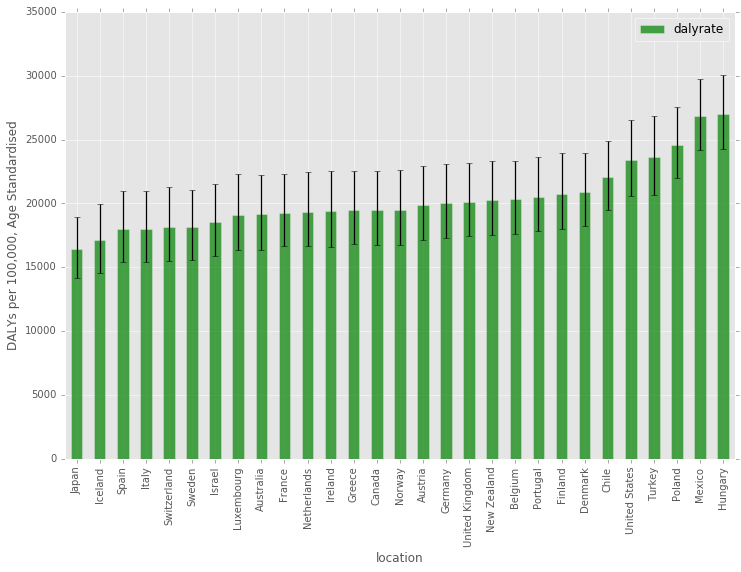# Trends Over Time¶

There is a pretty clear downward trend for most countries over this time period, although the slopes of countries like Turkey are much steeper.

In :
fig, ax = plt.subplots(figsize=(11,9))

countries = oecdgbd_df['location'].drop_duplicates()

for num, country in enumerate(countries):
x_vals = oecdgbd_df[(oecdgbd_df['location'] == country)].loc[:,'year']
y_vals = oecdgbd_df[(oecdgbd_df['location'] == country)].loc[:,'dalyrate']
#plt.scatter(x=x_vals, y=y_vals, color=colors[num], s=20, alpha=1.0, marker='o', linewidths=1) #, label=str(country)
#plot([1,2,3], [1,2,3], 'go-', label='line 1', linewidth=2)
if num % 4 == 0:
plt.plot(x_vals, y_vals,'-o', linewidth=2, label=str(country))
else:
plt.plot(x_vals, y_vals,'-o', linewidth=2, label='_nolegend_')

plt.xlabel('Year')
plt.ylabel('DALY per 100,000, Age Standardized')
plt.legend()
plt.show()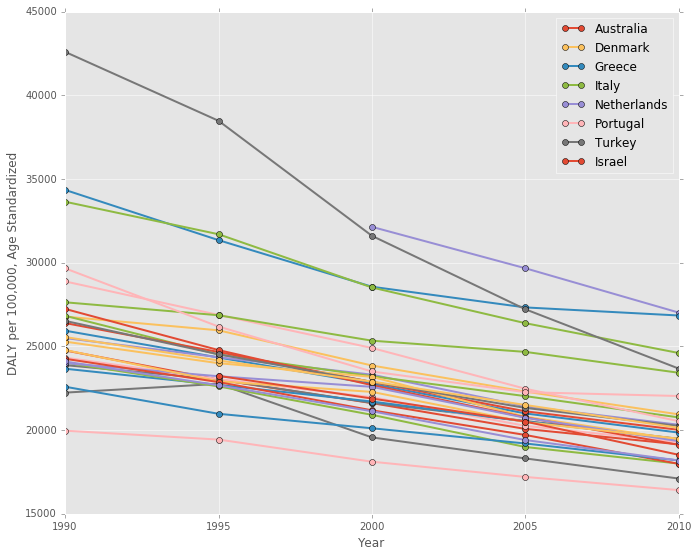# Health and Social Expenditure by Country¶

This next chart is a barplot, showing the social and health expenditure by country. It's ordered by the total social expenditure, descending. The US social services spending in on par with other countries, but the total health spending sticks out above most others.

In :
#Take a look at stacked bar of healthcare, ordered by total expenditure

fig = plt.figure() # Create matplotlib figure
ax = fig.add_subplot(111) # Create matplotlib axes
width = 0.5

#Sort by social_expenditure
oecdgbd2010_df.sort_values(by='social_exp',ascending=False)[['location','social_exp', 'health_exp']].plot.bar(
x='location', stacked=True, ax=ax, width=width, color=('green', 'lightgreen'),figsize=(12,8),alpha=0.9)

ax.set_ylabel('Per Capita Expenditure (2010 USD, PPP)')

plt.show()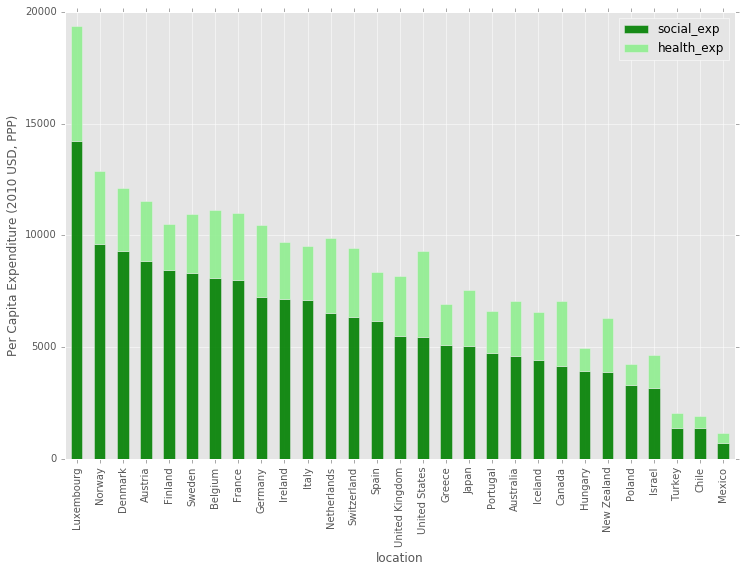# A Different Perspective¶

Next, here is another way to look at the above bar chart. This time, countries are ordered by their DALY burden on the x-axis, and the y-axis shows social and health expenditure. It's pretty striking how badly the United States is doing in health outcomes given the amount that it spends on health and social services. Also, it's interesting to note that Japan, Iceland, Israel, and a number of other countries spend less combined than the US with much better outcomes, so spending alone doesn't result in good health outcomes.

Are these differences due to our genetics/cultural norms/other social determinants in the US, or are we just really inefficient in the way we spend our money?

In :
fig, ax = plt.subplots(figsize=(12,8))

#http://matplotlib.org/1.3.0/api/axes_api.html#matplotlib.axes.Axes.vlines
#http://matplotlib.org/1.3.0/api/collections_api.html#matplotlib.collections.LineCollection
#Difference is the healt_exp, mimics stacked effect
ax.vlines(x = oecdgbd2010_df['dalyrate'], ymin=0, ymax = oecdgbd2010_df['total_exp'],
colors='lightgreen', linewidths=6, alpha=0.9, label='Health Expenditure')
ax.vlines(x = oecdgbd2010_df['dalyrate'], ymin=0,  ymax = oecdgbd2010_df['social_exp'],
colors='green', linewidths=6, alpha=0.7, label='Social Expenditure')

ax.set_xlabel('DALY per 100,000, Age Standardized')
ax.set_ylabel('Per Capita Expenditure, Social and Health (2010 USD, PPP)')

A = oecdgbd2010_df['dalyrate']
B = oecdgbd2010_df['total_exp']
C = oecdgbd2010_df['location']
D = range(len(C))

#print A, B, C, D

for a,b,c,d in zip(A, B, C, D):
if d % 1 == 0: #Annotate every n
ax.annotate('%s' % c, xy=(a,b), textcoords='data', rotation=90) #xy=(a,b+30)

plt.show()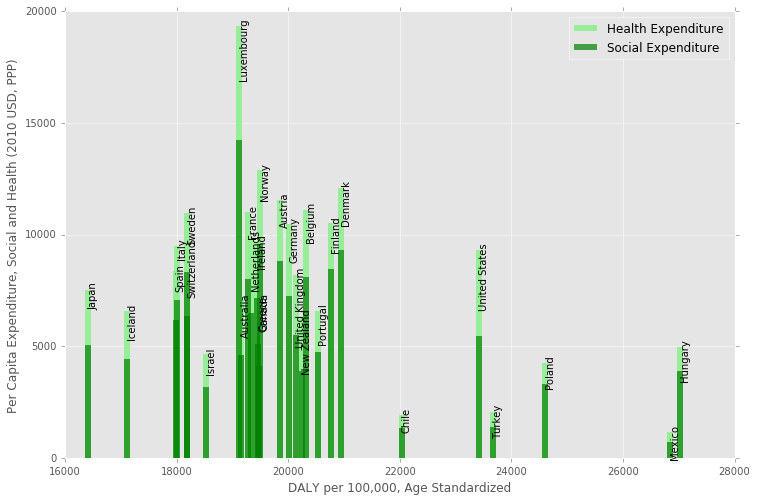Finally, here is a scatterplot of health versus social services expenditure. Aside from Luxembourg, the US has the highest health spending, but has much lower social services spending than most Western European countries.

In :
fig, ax = plt.subplots(figsize=(11,9))  #figsize=(12,10)

plt.scatter(x=oecdgbd2010_df['health_exp'], y=oecdgbd2010_df['social_exp'],
marker='o', alpha=0.9, label='_nolegend_')

ax.set_xlim(0,6000)
ax.set_ylim(0, 16000)
#s=20

# points linearlyd space on lstats
x = pd.DataFrame({'line': np.linspace(0, 16000, 100)})

plt.plot(x, x, 'b--', alpha=0.9, label='Equal')

A = oecdgbd2010_df['health_exp']
B = oecdgbd2010_df['social_exp']
C = oecdgbd2010_df['location']
D = range(len(C))

for a,b,c,d in zip(A, B, C, D):
if d % 1 == 0: #Annotate every n
ax.annotate('%s' % c, xy=(a,b), textcoords='data')

plt.xlabel('Per Capita Health Expenditure (2010 USD, PPP)')
plt.ylabel('Per Capita Social Expenditure (2010 USD, PPP)')

plt.legend()

plt.show()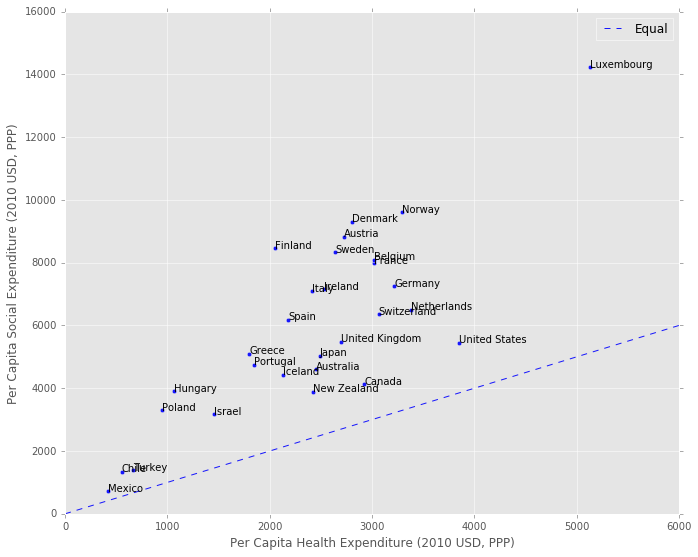# How are these variables related?¶

Pandas has a cool plotting function called a scatter matrix, where it will show you scatter plots of each variable against the others in your dataframe. In addition, it shows the Kernel Density Estimate (KDE) on the diagonal, which is an estimate of the Probability Density Function of each variable. This gives you a quick view of all the data, and potential distribution issues to look out for in the regression.

It looks like both the dalyrate and gdp_capita variables have positive skews based on their KDE, so it might make sense to normalize these variables using a log or some other correction.

In :
#Import scatter matrix function:
from pandas.tools.plotting import scatter_matrix

# Copy dataframe
model_df = oecdgbd_df.copy()

#Not sure 0 expenditure is a reliable number.
#Remove Slovenia, with value of 0 social_exp for 1995, and Chile, with 0 health_exp in 1990
model_df = model_df[(model_df['social_exp'] != 0 ) & (model_df['health_exp'] != 0 )]

scatter_matrix(model_df[['dalyrate', 'gdp_cap', 'social_exp', 'health_exp']],
alpha=1.0, figsize=(11, 10), diagonal='kde')  #diagonal='kde' or 'hist'

Out:
array([[<matplotlib.axes._subplots.AxesSubplot object at 0x10aed2fd0>,
<matplotlib.axes._subplots.AxesSubplot object at 0x10c021f50>,
<matplotlib.axes._subplots.AxesSubplot object at 0x10c0b0690>,
<matplotlib.axes._subplots.AxesSubplot object at 0x10b7476d0>],
[<matplotlib.axes._subplots.AxesSubplot object at 0x10b70ce50>,
<matplotlib.axes._subplots.AxesSubplot object at 0x10aee1890>,
<matplotlib.axes._subplots.AxesSubplot object at 0x10b246710>,
<matplotlib.axes._subplots.AxesSubplot object at 0x10a8d3cd0>],
[<matplotlib.axes._subplots.AxesSubplot object at 0x10a88f7d0>,
<matplotlib.axes._subplots.AxesSubplot object at 0x109836290>,
<matplotlib.axes._subplots.AxesSubplot object at 0x10a873dd0>,
<matplotlib.axes._subplots.AxesSubplot object at 0x10a32efd0>],
[<matplotlib.axes._subplots.AxesSubplot object at 0x10a917dd0>,
<matplotlib.axes._subplots.AxesSubplot object at 0x10a49b110>,
<matplotlib.axes._subplots.AxesSubplot object at 0x10a307090>,
<matplotlib.axes._subplots.AxesSubplot object at 0x10a2100d0>]], dtype=object)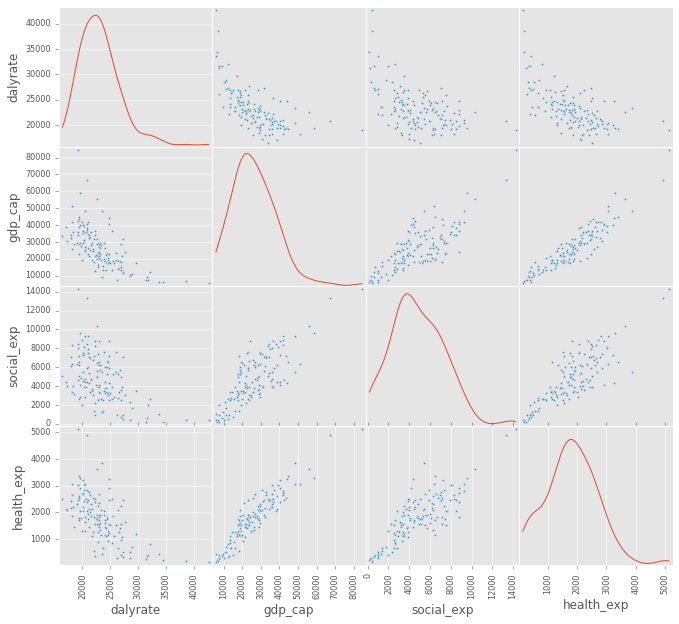# A Closer Look at Social Services and DALYs¶

Below is scatter of the social services expenditure against the DALY burden. There seems to be a clear log-linear, or even log-log relationship between the two, which makes sense given the Preston curve.

I also color coded each year to show the general shift down and to the right over time (better health, and more social services spending).

In :
fig, ax = plt.subplots(figsize=(11,9))  #figsize=(12,10)

# Points linearlyd space on lstats
x = pd.DataFrame({'social_exp': np.linspace(model_df['social_exp'].min(),
model_df['social_exp'].max(), 100)})

# 1st order polynomial
# Note: mse_resid is the sum of squared residuals divided by the residual degrees of freedom.
poly_1 = smf.ols(formula='dalyrate ~ 1 + social_exp', data=model_df, missing='drop').fit()

plt.plot(x, poly_1.predict(x), 'b-', label='Poly n=1 $R^2$=%.2f  RSE=%.2f' %
(poly_1.rsquared, np.sqrt(poly_1.mse_resid)), alpha=0.9)

#np.log is natural log:
#Log makes sense, given the Preston Curve: https://en.wikipedia.org/wiki/Preston_curve
log_1 = smf.ols(formula='dalyrate ~ 1 + np.log(social_exp)',
data=model_df, missing='drop').fit()

plt.plot(x, log_1.predict(x), 'y-', alpha=0.9, label='Log $R^2$=%.2f  RSE=%.2f' %
(log_1.rsquared, np.sqrt(log_1.mse_resid)) )

colors = ['#FB6A4A', '#6BAED6', '#9E9AC8', '#74C476', '#696969']

years = [1990, 1995, 2000, 2005, 2010]

for num, year in enumerate(years):
x_vals = model_df[(model_df['year'] == year)].loc[:,'social_exp']
y_vals = model_df[(model_df['year'] == year)].loc[:,'dalyrate']
plt.scatter(x=x_vals, y=y_vals, color=colors[num], s=20, alpha=1.0, marker='o', label=str(year))

plt.xlabel('Per Capita Social Expenditure (2010 USD, PPP)')
plt.ylabel('DALY per 100,000, Age Standardized')

plt.legend()
plt.show()

#print poly_1.summary()
#print log_1.summary()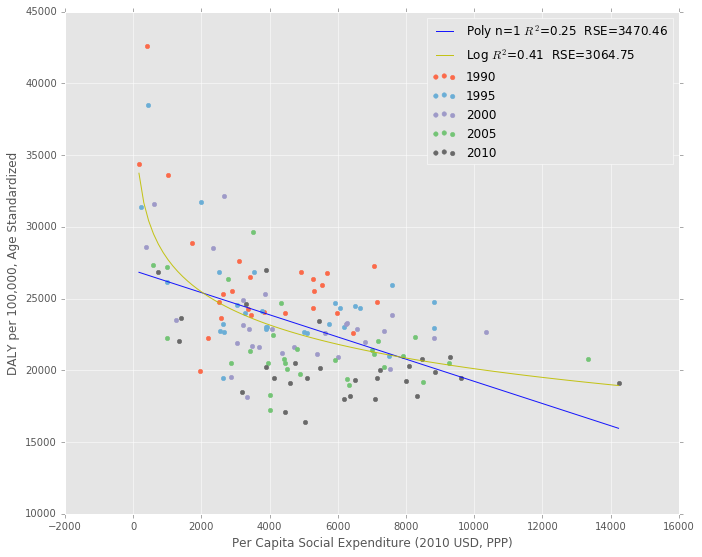# A Mixed Linear Model¶

It doesn't make sense to fit a Linear Regression to the entire dataset because the observations of each country over multiple years are not independent. The data are considered panel data, so either using General Estimating Equations or a Linear Mixed Effects Model is necessary to deal with interdependent measures. A good overview of the differences between the two is available here.

The authors of the original paper  decided to use a Mixed Linear Model, so that is what I'll use as well. Here is the description of their methods:

We used standard descriptive analyses to characterise the percentage of GDP in each country that was spent on health services, social services and the ratio of social expenditures to health expenditures, using 2005 data. In addition, we estimated a series of mixed-effects models with the pooled data over 11 years and 29 countries to examine the correlation of health service expenditures and the five outcomes, of social services expenditures and the five outcomes, and of the ratio of social to health service expenditures, adjusted for health expenditures, and the five outcomes. We also examined the interaction of social expenditures and health expenditures. In each model, we included the logarithm of GDP per capita measured in US dollars adjusted for purchasing power parity, and we allowed the intercept and the expenditure variables to vary randomly over countries. To account for heteroskedasticity, we estimated the residual errors inde- pendently for each country.

I don't have all the data from their study, and don't know enough about statistics to reproduce it in it's entirety, but I'll at least run the linear mixed effects regression of the DALY rate on per capita health, social services and GDP variables using Python Statsmodels.

Below, I take the natural log of the dalyrate variable to prevent heteroscedacity, and also take the natural log of the rest of the variables to make it easier to interpret the coefficients. I allow intercepts to vary randomly across the countries, and allow the slopes to vary randomly for the social and health expenditure variables. For more on how this model works, see the explanation in Appendix A.

In :
mixed_1 = smf.mixedlm(formula='np.log(dalyrate) ~ 1 + np.log(social_exp) + np.log(health_exp) + np.log(gdp_cap)',
data=model_df, missing='drop', groups='location',
re_formula='~ 1 + np.log(social_exp) + np.log(health_exp)').fit()

print mixed_1.summary()

                          Mixed Linear Model Regression Results
========================================================================================
Model:                      MixedLM         Dependent Variable:         np.log(dalyrate)
No. Observations:           141             Method:                     REML
No. Groups:                 29              Scale:                      0.0004
Min. group size:            3               Likelihood:                 232.0710
Max. group size:            5               Converged:                  Yes
Mean group size:            4.9
----------------------------------------------------------------------------------------
Coef.  Std.Err.   z    P>|z| [0.025 0.975]
----------------------------------------------------------------------------------------
Intercept                                     13.408    0.162 82.686 0.000 13.090 13.726
np.log(social_exp)                            -0.080    0.029 -2.735 0.006 -0.137 -0.023
np.log(health_exp)                            -0.046    0.034 -1.351 0.177 -0.112  0.021
np.log(gdp_cap)                               -0.234    0.026 -9.005 0.000 -0.285 -0.183
Intercept RE                                   0.454   11.343
Intercept RE x np.log(social_exp) RE          -0.011    1.163
np.log(social_exp) RE                          0.005    0.218
Intercept RE x np.log(health_exp) RE          -0.047    1.438
np.log(social_exp) RE x np.log(health_exp) RE -0.004    0.205
np.log(health_exp) RE                          0.012    0.329
========================================================================================


/Users/psthomas/miniconda2/envs/datascience/lib/python2.7/site-packages/statsmodels/regression/mixed_linear_model.py:1717: ConvergenceWarning: The MLE may be on the boundary of the parameter space.
warnings.warn(msg, ConvergenceWarning)


(Note that the above ConvergenceWarning is probably due to the fact that some of the coefficients are very small)

# Results¶

Overall, social expenditure and GDP per capita have significant and negative associations with the DALY burden at the 5% level. Becuase I took the natural log of both the independent and dependent variables, the coefficients can be interpreted as the percent change in DALY burden for each 1% change in the independent variable. So, in this case, a 1% increase in GDP per capita is associated with a -0.234% decline in DALY burden. The effect size for social expenditure is about a third that of GDP, at a -0.08% decline.

Note that these relationships aren't neccessarily causative. Whether GDP growth causes better health outcomes is actually still a pretty controversial question according to my analysis. It's possible that better health causes GDP growth, or there is a third factor like good institutions that causes both.

I'm not very confident in the results of this regression for a few reasons:

1. This is the first time I've done a regression analysis, and most of my background is from a chapter I read on the subject a week ago.
2. The results seem kind of fragile, and different data transforms and interaction effects can change the results pretty easily.
3. I'm not sure if I should include the year as a predictor as well.
4. I don't know how to check for heteroscedacity in the residuals, as statsmodels doesn't have a simple method for doing so with a mixed linear model.
5. There also aren't any easy tests for collinearity with the mixed linear model, so I'm not sure how to test for that issue.

Regardless of the above problems, the relationship between GDP and health outcomes is pretty constant between models, so I am fairly confident that association is significant. This fits with the narrative that there are some social determinants like GDP growth that are very important for health and that social or health services expenditures might not be sufficient replacements for the effects of growth on health.

When it comes to the policy implications, though, everyone and their mother seems to want to increase long term economic growth. But slow growth in the West seems to be a very tough problem to crack. So, in practice, increasing social services spending in a cost effective way might be the most actionable policy advice for improving health.